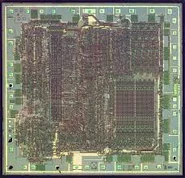69 PagesThe Instruction set below can be downloaded in Word format from the Crib Sheets page.

 Instruction Syntax Description ADC ADC   HL,ss Add with carry register pair ss to HL. ADC   A,s Add with carry operand s to accumulator. ADD   A,n Add value n to accumulator. ADD   A,r Add register r to accumulator. ADD   A,(HL) Add location (HL) to acccumulator. ADD   A,(IX/IY+d) Add location (IX or IY +d) to accumulator. ADD   HL,ss Add register pair ss to HL. ADD   IX,pp Test bit b of location (IX+d). BIT BIT   b,(IY+d) Test bit b of location (IY+d). BIT   b,r Test bit b of register r. CALL CALL  cc,nn Call subroutine at location nn if condition CC is true. CCF CCF Complement carry flag. CP CP    s Compare operand s with accumulator. CPD CPD Compare location (HL) and acc., decrement HL and BC CPDR CPDR Perform a CPD and repeat until BC=0. CPI CPI Compare location (HL) and acc., incr HL, decr BC. CPIR CPIR Perform a CPI and repeat until BC=0. CPL CPL Complement accumulator (1's complement). DAA DAA Decimal adjust accumulator. DEC DEC   m Decrement operand m. DEC   IX/IY Decrement IX/IY DEC   ss Decrement register pair ss. DI DI Disable interrupts. DJNZ DJNZ  e Decrement B and jump relative if B=0. EI EI Enable interrupts. EX EX    (SP),HL Exchange the location (SP) and HL. EX    (SP),IX/IY Exchange the location (SP) and IX/IY EX    AF,AF' Exchange the contents of AF and AF'. EX    DE,HL Exchange the contents of DE and HL. EXX EXX Exchange the contents of BC,DE,HL with BC',DE',HL'. HALT HALT Halt computer and wait for interrupt. IM IM    0 Set interrupt mode 0. IM    1 Set interrupt mode 1. IM    2 Set interrupt mode 2. IN IN    A,(n) Load the accumulator with input from device n. IN    r,(c) Load the register r with input from device (C). IN    (HL) Increment location (HL). INC INC   IX/IY Increment IX./IY INC   (IX/IY+d) Increment location (IX/IY+d). INC   r Increment register r. INC   ss Increment register pair ss. IND IND (HL)=Input from port (C). Decrement HL and B. INDR INDR Perform an IND and repeat until B=0. INI INI (HL)=Input from port (C). HL=HL+1. B=B-1. INIR INIR Perform an INI and repeat until B=0. JP JP    (HL) Unconditional jump to location (HL). JP    (IX) Unconditional jump to location (IX). JP    (IY) Jump to location nn if condition cc is true. JP    cc,nn Jump to location nn if condition cc is true. JR JR    e Unconditional jump relative to PC+e. JR    NC,e Jump relative to PC+e if carry=0. JR    NZ,e Jump relative to PC+e if non zero (Z=0). JR    Z,e Jump relative to PC+e if zero (Z=1). LD LD    A,(BC) Load accumulator with location (BC). LD    A,(DE) Load accumulator with location (DE). LD    A,I Load accumulator with I LD    A,(nn) Load accumulator with location (nn) LD    A,R Load accumulator with R. LD    (dd),A Load location register pair with accumulator. LD    dd, nn Load register pair dd with nn. LD    dd,(nn) Load register pair dd with location (nn). LD    HL,(nn) Load HL with location (nn). LD    (HL),r Load location (HL) with register r. LD    I,A Load I with accumulator. LD    IX/IY,nn Load IX/IY with value nn. LD    IX/IY,(nn) Load IX/IY with location (nn). LD    (IX/IY+d),n Load location (IX/IY+d) with n. LD    (IX/IY+d),r Load location (IX/IY+d) with register r. LD    (nn),A Load location (nn) with accumulator. LD    (nn),dd Load location (nn) with register pair dd. LD    (nn),IX/IY Load register r with location (IX/IY+d). LD    r,n Load register r with value n. LD    r,r' Load register r with register r'. LD    SP,HL Load SP with HL. LD    SP,IX/IY Load SP with IX. LDD LDD Load location (DE) with location (HL), decrement DE,HL,BC. LDIR LDIR Perform an LDI and repeat until BC=0. NEG NEG Negate accumulator (2's complement). NOP NOP No operation. OR    s Logical OR of operand s and accumulator. OTDR OTDR Perform an OUTD and repeat until B=0. OTIR OTIR Perform an OTI and repeat until B=0. OUT OUT   (C),r Load output port (C) with register r. OUT   (n),A Load output port (n) with accumulator. OUTD OUTD Load output port (C) with (HL), decrement HL and B. OUTI OUTI Load output port (C) with (HL), incr HL, decr B. POP POP   IX/IY Load IX/IY with top of stack. POP   qq Load register pair qq with top of stack. PUSH PUSH  IX/IY Load IX/IY onto stack. PUSH  qq Load register pair qq onto stack. RES RES   b,m Reset bit b of operand m. RET RET Return from subroutine. RET cc Return from subroutine if condition cc is true. RETI RETI Return from interrupt. RETN RETN Return from non-maskable interrupt. RL    m Rotate left through operand m. RLA RLA Rotate left accumulator through carry. RLC RLC   (HL) Rotate location (HL) left circular. RLC   (IX/IY+d) Rotate location (IX/IY+d) left circular. RLC   r Rotate register r left circular. RLCA RLCA Rotate left circular accumulator. RLD RLD Rotate digit left and right between accumulator and (HL). RR RR    m Rotate right through carry operand m. RRA RRA Rotate right accumulator through carry. RRC m RRC m Rotate operand m right circular. RRCA RRCA Rotate right circular accumulator. RRD RRD Rotate digit right and left between accumulator and (HL). RST RST p Restart to location p. SBC   A,s Subtract operand s from accumulator with carry. SBC   HL,ss Subtract register pair ss from HL with carry. SCF SCF Set carry flag (C=1). SET SET   b,(HL) Set bit b of location (HL). SET   b,(IX/IY+d) Set bit b of location (IX/IY+d). SET   b,R Set bit b of register r. SLA SLA m Shift operand m left arithmetic. SRA SRA m Shift operand m right arithmetic. SRL SRL m Shift operand m right logical. SUB SUB s Subtract operand s from accumulator. XOR XOR s Exclusive OR operand s and accumulator.
Community content is available under CC-BY-SA unless otherwise noted.